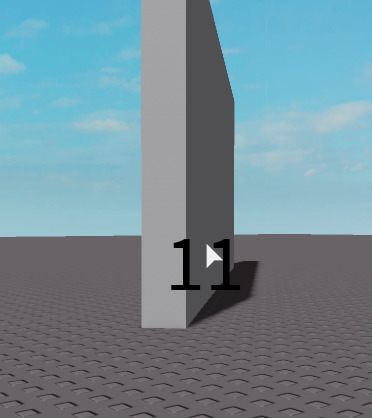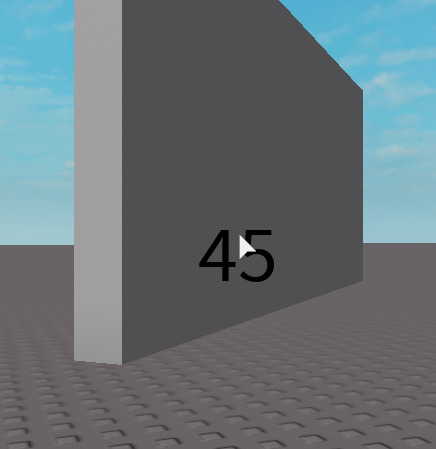# Degrees between vectors

Hello devs!
I doing bullets script for my game and i wanted to do rickoshet when degrees between old and new vectors is >45 but i got a problem, i tryed to find some formules about this and i find it but this dont work for me, maybe i doing something wrong?

``````function understandRickoshet(Vector,Normal)
local NewVector = (Vector-(2*Vector:Dot(Normal)*Normal))
print("rickoshet")
end
end
``````

Numbers which this function returns sometimes looks like true but then its brokes.
Sorry for my grammar

First, you can simplify a bit with `Unit` and `Dot`:

``````-- I'm assuming Normal is already a unit vector
local NewVector = Vector - 2*Vector:Dot(Normal)*Normal
local angle = math.acos(Vector.Unit:Dot(Normal)) -- in radians
``````

Also, you’re checking if the angle is <45 degrees because of your `180-` part, is that what you want? I would think that ricochets happen when the reflection and the incidence are large angles:

``````if math.deg(angle) > 45 then
print("Ricochet")
end
``````

Maybe these will fix your issues

1 Like

robloxapp-20210224-2100027.wmv (2.5 МБ)
Same problem

``````function understandRickoshet(Vector,Normal)
local NewVector = Vector - 2*Vector:Dot(Normal)*Normal
script.ScreenGui.TextButton.Text = "Rickoshet"
else
script.ScreenGui.TextButton.Text = "No Rickoshet"
end
end
``````

How do you call this function? What are `Vector` and `Normal`?

``````local Reflection = BulletDirection - 2 * BulletDirection:Dot(SurfaceNormal) * SurfaceNormal
``````

I cann this function when bullet hit something, Vector that’s bullet vector at hit moment and Normal is vector’s normal

``````CurObj,CurEndPos,CurNormal,CurMaterial = workspace:FindPartOnRayWithWhitelist(Ray.new(HP+Bullet.Vector*Bullet.PenetrationPower,-Bullet.Vector*Bullet.PenetrationPower),{HO})
if CurObj then
understandRickoshet(Bullet.Vector,CurNormal)
end
``````

That’s start of shooting trough walls script

This already works like this because BulletDirecton is “Vector” and SurfaceNormal its “Normal”

``````local angle = math.acos(Vector.Unit:Dot(Normal))
``````

should be

``````local angle = math.acos(Vector.Unit:Dot(NewVector.Unit))
``````

But that would just cut the angle in half it wouldn’t break it.

Maybe the bullet is just penetrating the wall when you’re looking head-on and the raycast is skipped?

I tested this code which raycasts from the camera to the mouse and shows the angle and it seems to be working like I expect:

Expand to see code I used
``````local player = game.Players.LocalPlayer
local character = player.Character or player.CharacterAdded:Wait()
local mouse = game.Players.LocalPlayer:GetMouse()
local label = player.PlayerGui.ScreenGui.TextLabel

local function understandRickoshet(Vector,Normal)
local NewVector = Vector - 2*Vector:Dot(Normal)*Normal
end

game:GetService("RunService").Stepped:Connect(function()
local ray = Ray.new(mouse.UnitRay.Origin, mouse.UnitRay.Direction * 999)
local CurObj,CurEndPos,CurNormal,CurMaterial = workspace:FindPartOnRayWithIgnoreList(ray, {character})
if CurObj then
understandRickoshet(ray.Direction,CurNormal)
end
end)
``````

Vectors are close to aligned when I just graze the surface:Increase as I move to more perpendicular:Up to 180 degrees in the middle:

You can try using the Lua debugger to step through your code and see if things make sense to you. Or add some print statementsYou was right i just sended back normal from shooting trough walls scriptlmao, also thanks